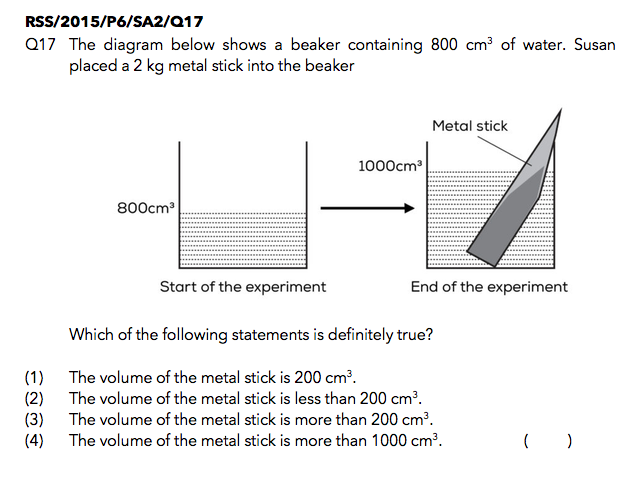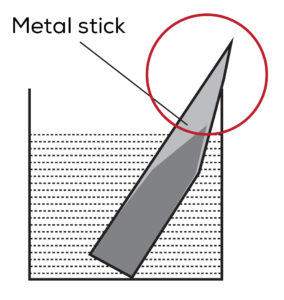Last Updated On

Matter is a topic that students learn either in P3 or P4. Students are required to know and are often tested on the properties of matter in examinations.

A common question that students tend to ask when they are first exposed to this topic is:

### “What is matter?”

#### “Matter is anything that has mass and occupies space.”

Based on this definition, there are 2 important properties that have to be fulfilled in order to be classified as matter:

1. Has mass
2. Occupies space

What Does It Mean To Have Mass?

1. Does it give a reading when you place it on a weighing scale?
2. Does it feel heavier if you carry more of it?

What Does It Mean To Occupy Space?

1. Does it have to be removed in order for another object to take its place?
2. Does it take up space when placed in a container or location?
3. If the answers to the above questions are “yes”, the object is classified as matter. However, if the answers to the questions are “no”, the object is classified to be a non-matter.

With this understanding, we will now focus on the property “Matter occupies space” in this post.

All matter occupies space but how do we know how much space different matter occupy?

We measure its volume.

### “Volume is the amount of space that a body or object occupies.”

The volume of regular objects, such as a cube, can be calculated by multiplying its length, breadth and height.

For irregular objects, such as a stone, we measure its volume using water and a measuring cylinder/beaker.

## Let’s Take A Look At This QuestionSource: Red Swastika School – 2015 P6 Preliminary Science Examination Paper [Q17]

To measure the volume of an irregular object, like this metal stick, we use a beaker of water.

When the metal stick is placed into the beaker of water with a volume of 800 cm3, the new volume of water becomes 1000 cm3.In the question, a 2 kg metal stick has been placed into a beaker filled with 800 cmof water.

The purpose of this is to test if students are able to find out the volume of the object.

On seeing this, students will quickly conclude by taking the (new volume of the water + metal stick) – (old volume of water) i.e. 1000-800 = 200. They will choose option (1) as the answer, thinking that the volume of the metal stick is 200 cm3.

Unfortunately, examination questions are no longer as straightforward but tricky!

Students do not realise that the volume of the metal stick is not equal to 200 cm3 as the stick is not fully submerged in water. There is a small part that is above the surface of the water as shown in the picture below.In order to measure the full volume of an object, the object needs to be fully submerged in water. As such, the 200 cm3 calculated earlier would only be the part where the object is submerged in water, excluding the part that is circled in the picture shown above.

Hence, the volume of the whole metal stick would have to be (200cm3  + the volume of the part circled). The option that is definitely true would therefore be:

(3) The volume of the metal stick is more than 200 cm3.

## In Conclusion

I hope this article has helped you gain a better understanding of this property of matter, “Matter occupies space”.

These days, examination questions are no longer that straight forward, so your child needs to be extra careful when analysing these questions.